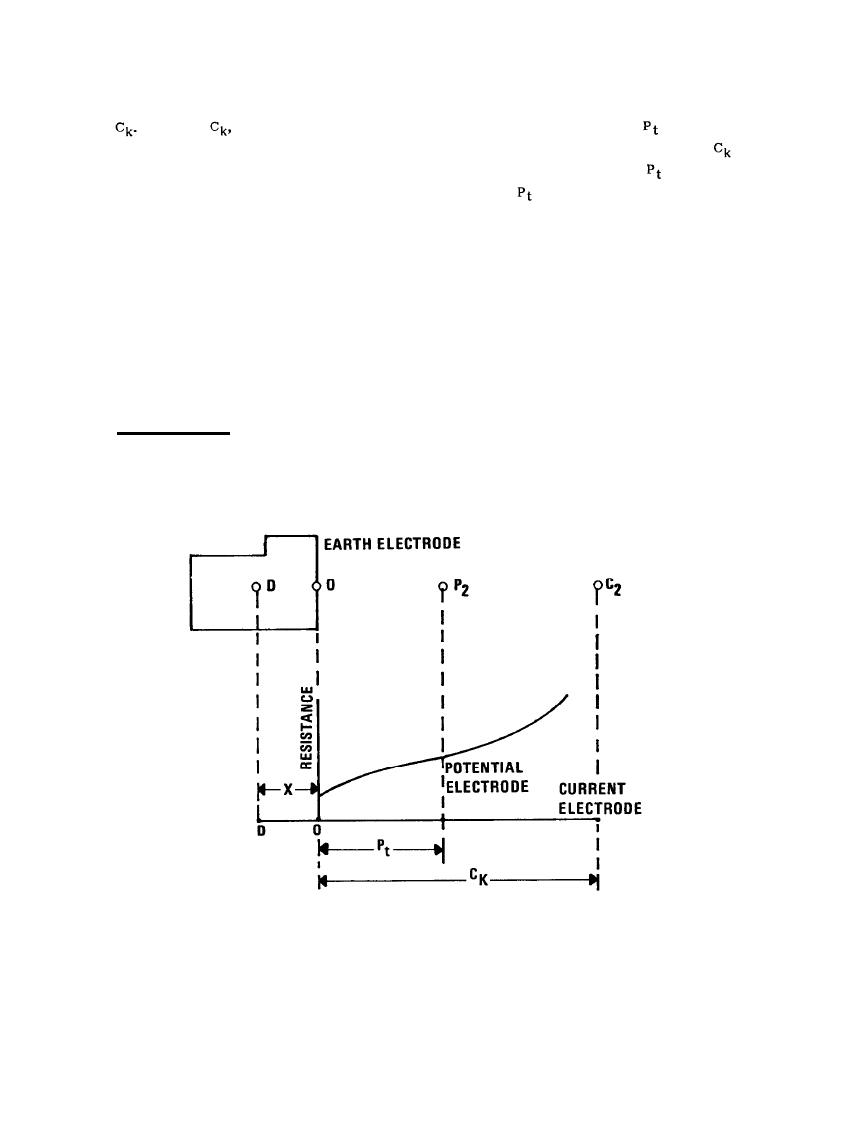Figure 2-19. Earth Resistance Curve Applicable to Large Earth Electrode SubsystemsCustom SearchMIL-HDBK-419A
To determine the true resistance of the earth electrode, X is allowed to assume convenient increments from
zero to
For each
the value of measured resistance corresponding to the resultant
(calculated with
Equation 2-50) is read from the curves of Figure 2-18 and plotted against X. For example, if X and
both
equal 305 M (1000 feet), considering only the right hand curve in Figure 2-18, the value of
is 240, and R is
0.08 ohms. Next let X be 244 m (800 feet). The corresponding value of
is 96 m (316 feet) and r is 0.1 ohms.
In this manner, estimates of the 62 percent values can be taken from Figure 2-18 and replotted as "true"
resistance versus X, as shown in Figure 2-20. At the region of intersection of the curves in Figure 2-20, the
value of X = 122 m (400 feet) corresponds to the electrical center of the electrode, and the corresponding value
of resistance (0.13 ohms) is the true value of resistance-to-earth of the electrode system. It is recommended
that the distance to the current probe, "C", from the point of connection to the earth electrode, "O", (see
Figure 2-19) be between one and two times the length of the longest side of the electrode system.
Furthermore, failure to obtain a well defined region of intersection of the curves can result if the probe
measurements are not taken on a radial from the electrical center, in that case, new probe directions will be
required.
2.7.2.3 Test Equipments. Test equipments are presently available  which will permit the accurate
measurement of ground resistances of earth electrode subsystems from 0.01 to 20,000 ohms and above. Most
equipments used in conducting these measurements are designed to utilize ground test currents other than dc or
60 Hz to avoid or eliminate the effects of stray ac or dc currents in the earth.
Figure 2-19. Earth Resistance Curve Applicable to Large Earth Electrode Subsystems
2-45Integrated Publishing, Inc.